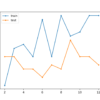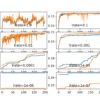# Archive | Deep Learning Performance## How to Control Neural Network Model Capacity With Nodes and Layers

The capacity of a deep learning neural network model controls the scope of the types of mapping functions that it is able to learn. A model with too little capacity cannot learn the training dataset meaning it will underfit, whereas a model with too much capacity may memorize the training dataset, meaning it will overfit […]## Framework for Better Deep Learning

Modern deep learning libraries such as Keras allow you to define and start fitting a wide range of neural network models in minutes with just a few lines of code. Nevertheless, it is still challenging to configure a neural network to get good performance on a new predictive modeling problem. The challenge of getting good […]## How to Improve Performance With Transfer Learning for Deep Learning Neural Networks

An interesting benefit of deep learning neural networks is that they can be reused on related problems. Transfer learning refers to a technique for predictive modeling on a different but somehow similar problem that can then be reused partly or wholly to accelerate the training and improve the performance of a model on the problem […]Training a neural network can become unstable given the choice of error function, learning rate, or even the scale of the target variable. Large updates to weights during training can cause a numerical overflow or underflow often referred to as “exploding gradients.” The problem of exploding gradients is more common with recurrent neural networks, such […]## How to use Data Scaling Improve Deep Learning Model Stability and Performance

Deep learning neural networks learn how to map inputs to outputs from examples in a training dataset. The weights of the model are initialized to small random values and updated via an optimization algorithm in response to estimates of error on the training dataset. Given the use of small weights in the model and the […]## How to Use Greedy Layer-Wise Pretraining in Deep Learning Neural Networks

Training deep neural networks was traditionally challenging as the vanishing gradient meant that weights in layers close to the input layer were not updated in response to errors calculated on the training dataset. An innovation and important milestone in the field of deep learning was greedy layer-wise pretraining that allowed very deep neural networks to […]## How to Choose Loss Functions When Training Deep Learning Neural Networks

Deep learning neural networks are trained using the stochastic gradient descent optimization algorithm. As part of the optimization algorithm, the error for the current state of the model must be estimated repeatedly. This requires the choice of an error function, conventionally called a loss function, that can be used to estimate the loss of the […]## Loss and Loss Functions for Training Deep Learning Neural Networks

Neural networks are trained using stochastic gradient descent and require that you choose a loss function when designing and configuring your model. There are many loss functions to choose from and it can be challenging to know what to choose, or even what a loss function is and the role it plays when training a […]## Understand the Impact of Learning Rate on Neural Network Performance

Deep learning neural networks are trained using the stochastic gradient descent optimization algorithm. The learning rate is a hyperparameter that controls how much to change the model in response to the estimated error each time the model weights are updated. Choosing the learning rate is challenging as a value too small may result in a […]## How to Configure the Learning Rate When Training Deep Learning Neural Networks

The weights of a neural network cannot be calculated using an analytical method. Instead, the weights must be discovered via an empirical optimization procedure called stochastic gradient descent. The optimization problem addressed by stochastic gradient descent for neural networks is challenging and the space of solutions (sets of weights) may be comprised of many good […]